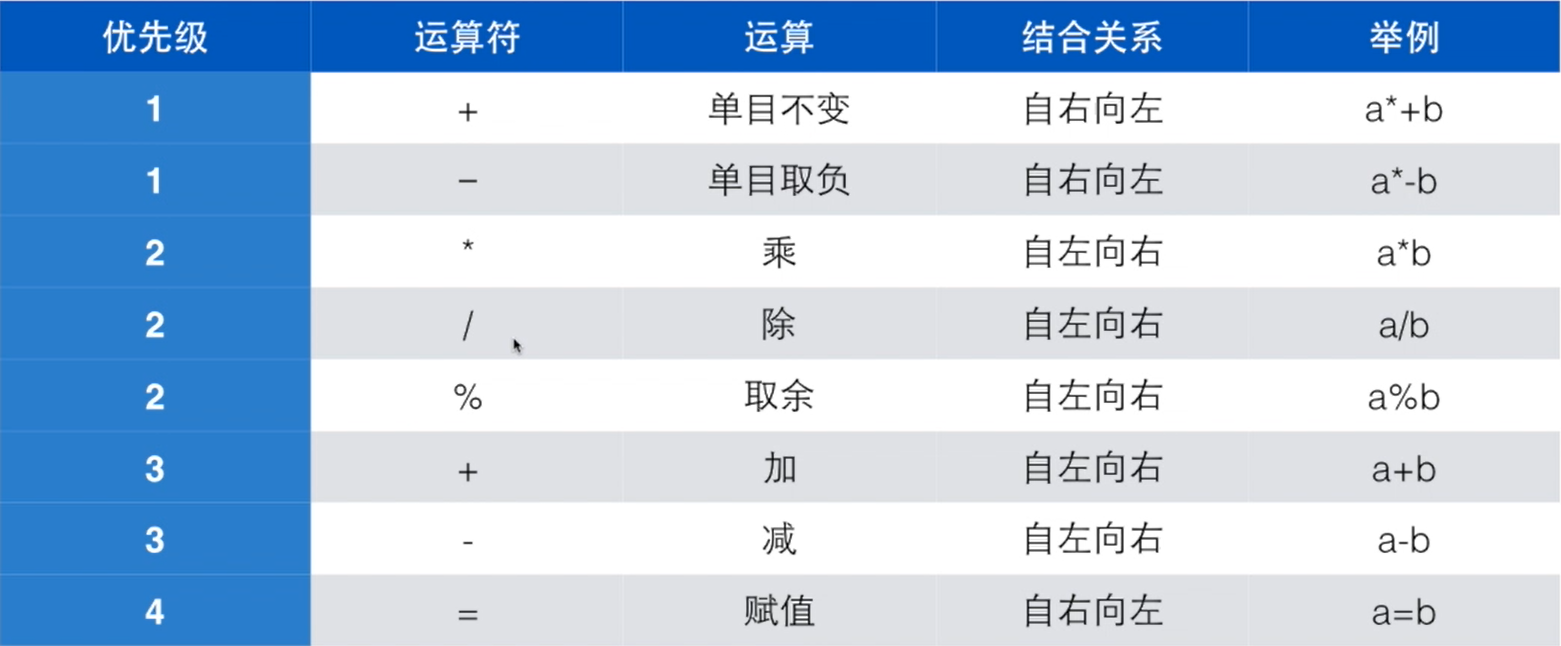``````#include <stdio.h>

int main()
{
printf("计算两个时间差");
int hour1,minute1;
int hour2,minute2;
scanf("%d %d",&hour1,&minute1);
scanf("%d %d",&hour2,&minute2);
int t1=hour1 * 60 + minute1;
int t2=hour2 * 60 + minute2;
printf("时间差为%d小时%d分\n",(t2-t1)/60,(t2-t1)%60);
return 0;
}``````
``````\$ cpp main.c -o main.ii
\$ cc main.ii -o main
\$ ./main

Program exited with status 0``````

``````#include <stdio.h>

int main()
{
int a;
int b;
scanf("%d %d",&a,&b);
double c= (a + b)/2.0;
printf("%d和%d的平均值是%f",a,b,c);
return 0;
}``````
``````\$ cpp main.c -o main.ii
\$ cc main.ii -o main
\$ ./main
2 3
2和3的平均值是2.500000Program exited with status 0``````双目运算符：在运算符左右有两个算子，如：5+6； 单目运算符：在运算符的右边有一个算子，如：-6。 ::: tip 单目运算符一般用于想要对某一个变量取负时，如想要取得变量a的相反数，取负操作是在变量a前加上一个单目运算符“-”。 单目运算优先级最高。 ::: 结合关系：相同优先级的运算中，除单目运算和赋值运算以外，均为自左向右。 ::: tip 单目运算符的算子一定出现在右边。 ::: ::: warning 避免“嵌入式赋值”

``````int a=6；
int b；
int c=1+(b=a);``````

“嵌入式赋值”不利于阅读且容易造成误解。 :::

``````a += 5 + 9
a = a + (5 + 9)
a /= b + 6
a = a / (b + 6)``````

``````count ++ ;
count += 1;
count = count + 1;``````

``````#include <stdio.h>

int main()
{
int a;
scanf("%d",&a);
printf("a++的值为%d\n",a++);
printf("a的值为%d\n",a);

printf("++a的值为%d\n",++a);
printf("a的值为%d\n",a);
return 0;
}``````
``````\$ cpp main.c -o main.ii
\$ cc main.ii -o main
\$ ./main
10
a++的值为10
a的值为11
++a的值为12
a的值为12
Program exited with status 0``````Suzhou
Lv1

17

4

0